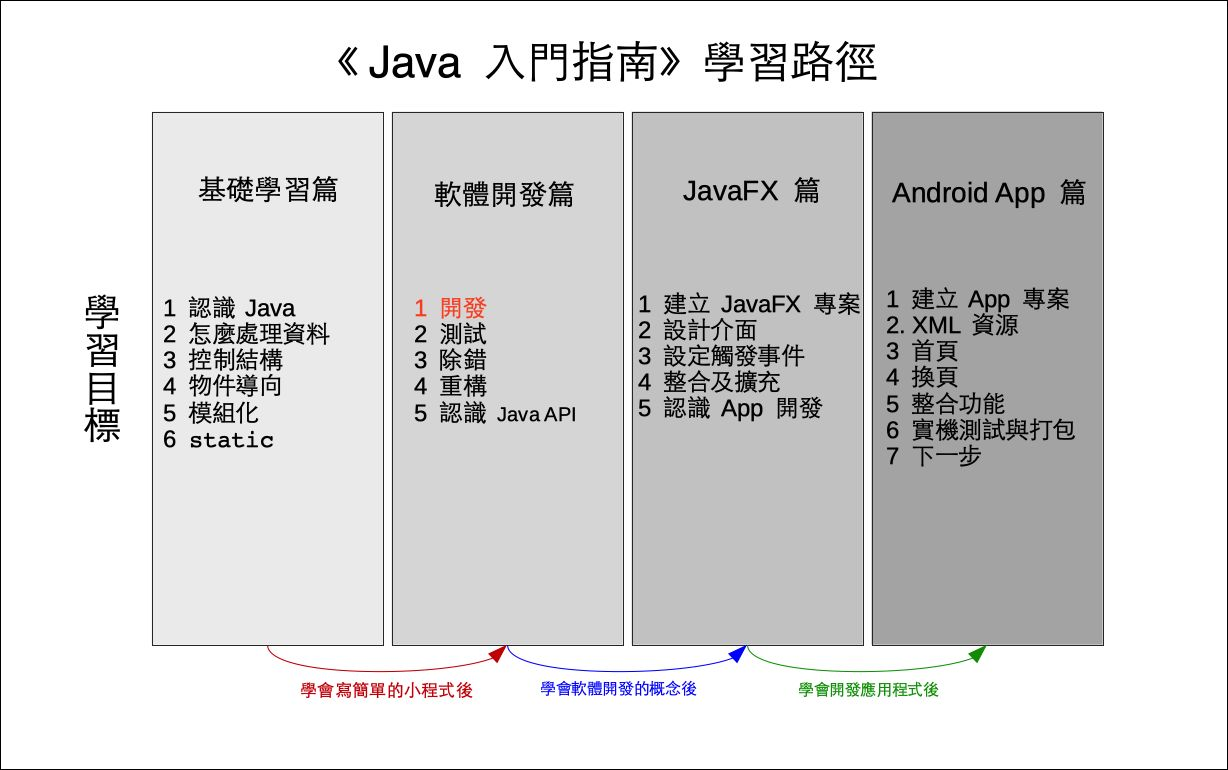# 單元 17 - 編碼與解碼

～～學習進度表～～There is no spoon.
×

 0 1 2 3 4 5 6 7 8 9 10 11 12 13 14 15 16 17 18 19 20 21 22 23 24 25 q z i r a j s b k t c l u d m v e n w f o x g p y h

``code_array = "qzirajsbktcludmvenwfoxgpyh"``

``"There is no spoon."``

``````// 編碼的方法
public String toEncode(String s) {
char[] cs = s.toCharArray();
int i, ci, d, m;
char r;
String rs = "";
Character cc;

for (i = 0; i < cs.length; i++) {
if (cs[i] >= 97 && cs[i] <= 122) {
ci = cs[i];
m = ci - 97;
cs[i] = code[m];
}
}

for (i = 0; i < cs.length; i++) {
cc = new Character(cs[i]);
rs = rs.concat(cc.toString());
}

return rs;
}``````

toEncode() 接收一個字串 s 當參數 (parameter) ，也回傳一個新字串 rss 就是要編碼的字串，而 rs 則是編碼過的字串。由於字串被設計成不可變的 (immutable) ，因此無法直接修改參數 s ，而要用一個新的字串變數。

``char[] cs = s.toCharArray();``

``````for (i = 0; i < cs.length; i++) {
if (cs[i] >= 97 && cs[i] <= 122) {
ci = cs[i];
m = ci - 97;
cs[i] = code[m];
}
}``````

``cs[i] = code[m];``

``````for (i = 0; i < cs.length; i++) {
cc = new Character(cs[i]);
rs = rs.concat(cc.toString());
}``````

``````// 解碼的方法
public String toDecode(String s) {
char[] cs = s.toCharArray();
int i, j;
char r;
String rs = "";
Character cc;

for (i = 0; i < cs.length; i++) {
if (cs[i] >= 97 && cs[i] <= 122) {
for (j = 0; j <= code.length; j++) {
if (cs[i] == code[j]) {
cs[i] = (char) (j + 97);
break;
}
}
}
}

for (i = 0; i < cs.length; i++) {
cc = new Character(cs[i]);
rs = rs.concat(cc.toString());
}

return rs;
}``````

toDecode()toEncode() 相似，同樣需要一個字串當參數，結果也回傳一個字串。

``````for (i = 0; i < cs.length; i++) {
if (cs[i] >= 97 && cs[i] <= 122) {
for (j = 0; j <= code.length; j++) {
if (cs[i] == code[j]) {
cs[i] = (char) (j + 97);
break;
}
}
}
}``````

 zejotydinsxchmrwbglqvafkpu There is no spoon. Titgt nl mr lwrrm. There is no spoon.array陣列
character字元
decoding解碼
encoding編碼
field屬性
immutable不可變的
index索引值
loop迴圈
nested loop巢狀迴圈
parameter參數
refactoring重構
string字串

1. The Java™ Tutorials: Declaring Classes
2. The Java™ Tutorials: Declaring Member Variables
3. The Java™ Tutorials: Defining Methods
4. The Java™ Tutorials: Providing Constructors for Your Classes
5. The Java™ Tutorials: Returning a Value from a Method
6. The Java™ Tutorials: Using the this Keyword
8. The Java™ Tutorials: Understanding Class Members
9. The Java™ Tutorials: Initializing Fields

1. 轉換表格利用字元陣列來儲存，字元陣列可用索引值存取元素，也就是字元。
2. 編碼時利用迴圈依序取得要編碼字串，先將編碼字串轉換成字元陣列，然後先判斷是否為英文小寫字母，如果是英文小寫字母就進行編碼。
3. 解碼用到巢狀迴圈，巢狀迴圈是指迴圈中還有另一個迴圈，第一層迴圈依序取得每一個解碼字元，第二層迴圈則是找出解碼字元在密碼表中的索引值，這個索引值也就是該字元在字母表中的索引值。

1. 要怎麼判斷一個字元是英文小寫字母或大寫字母？
2. 可以不用巢狀迴圈來解碼嗎？
3. 巢狀迴圈可以用一樣名稱的控制變數嗎？

1. 承接上一個單元的猜數字遊戲，將新專案寫在 Exercise1701 中，在遊戲迴圈中檢查使用者輸入的長度，若是長度不等於 4 就印出提示訊息進行下一輪。
2. 承上題，將新專案寫在 Exercise1702 中，把答案 answer 設定為 "0123456789" ，然後加入以下程式碼
``````List<String> answerList = new ArrayList<String>(Arrays.asList(answer.split("")));